# How to solve systems of linear equations on the SAT & ACT Math sectionsLinear equations are one of the most commonly tested parts of the Math sections of the SAT and ACT. Questions about lines can cover any difficulty level, from straightforward questions about slopes and intercepts to complicated questions that require us to apply linear functions to real-world problems.

Let’s jump into an application of linear equations: solving for multiple variables in a multi-equation system. First, let’s check out this sample SAT question: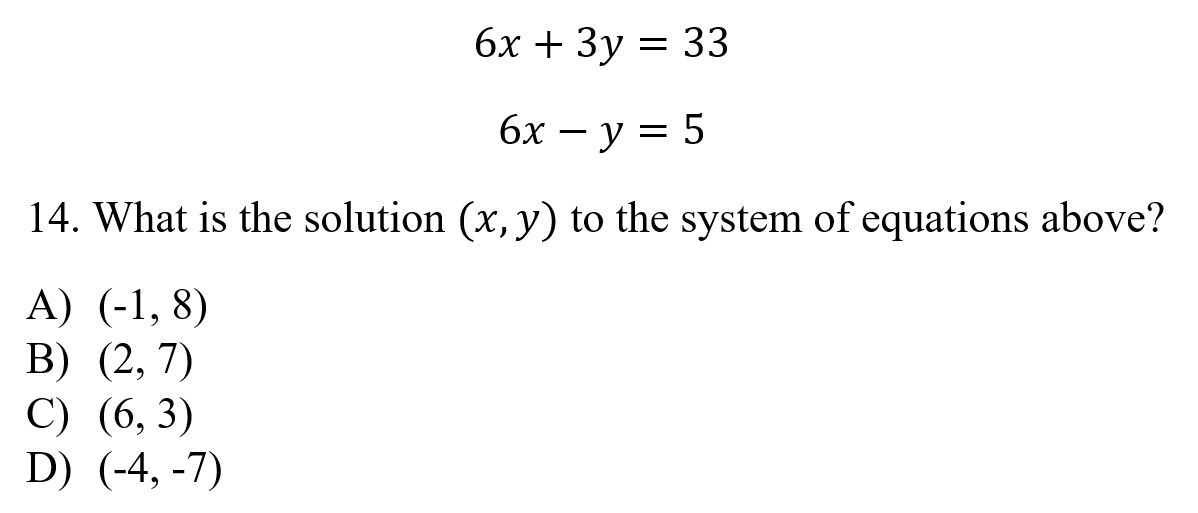This is a very common question type in which we are given the equations of two lines and are asked which coordinate pair satisfies both equations. If you see a question like this on the SAT or ACT, there are two equally valid techniques that will get you the correct solution. We’ll look at both, and you can choose which one to use on future questions.

#### Substitution

The first technique is the substitution technique. Here, we pick one equation and solve for a variable. I’m going to take the second equation and solve for y.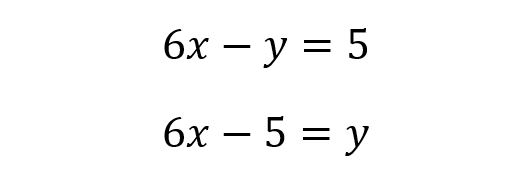Note: you should recognize this as the equation of a line in slope-intercept form. (Quiz yourself: what are the slope and y-intercept of this line?)

Next, we go back to the first equation, and wherever we see “y”, we substitute “6x – 5”. This gives us one equation in one variable: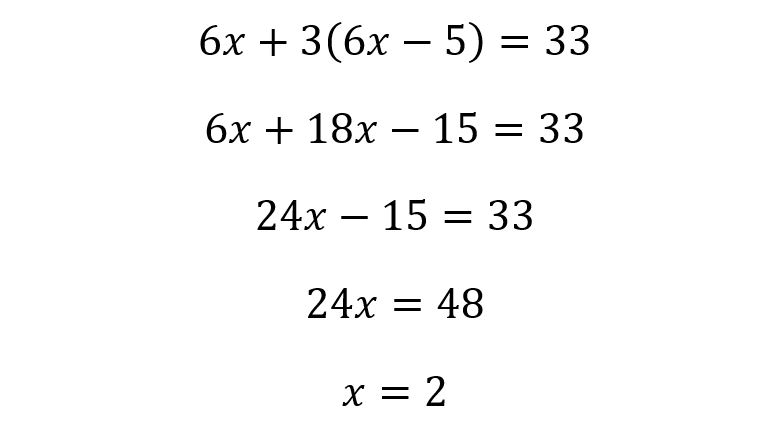We’re almost there. To find y, we simply plug x=2 back in to either of the two equations: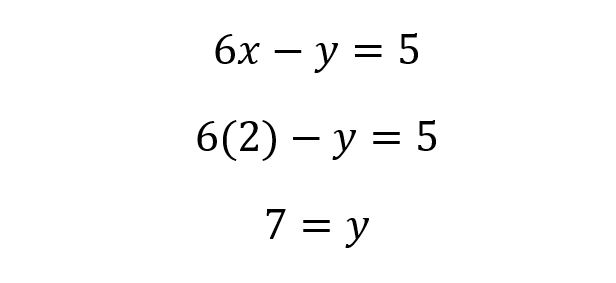With x being equal to 2 and y being equal to 7, our answer is (2, 7), or (B).

#### Elimination

The second technique for solving these problems is the elimination method. The goal of the elimination method is the same as that of the substitution method: to manipulate the equations so that we have one equation in one variable. But the elimination method does this in a much different way.

Take a look at the original two equations from the problem. Notice that the coefficient in front of the two x terms is the same: 6. In this case, we can subtract the entire second equation from the first equation, eliminating the x terms: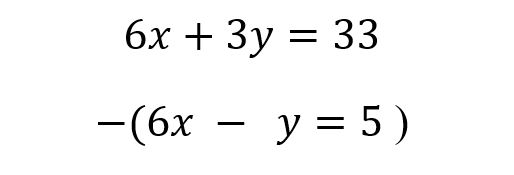Next, we simply subtract each term from the second equation from the first. 6x — 6x = 0, so the x terms are eliminated. 3y — (–y) = 4y, and 33 — 5 = 28.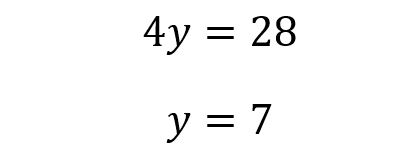The final step is the same as in the substitution method. We choose either equation and substitute y = 7, again finding that our correct answer is (2, 7).

Once you’ve mastered the basics, you’ll be able to apply them to a variety of problems. When you’re asked to find the coordinate pair that satisfies both equations, use whichever technique—substitution or elimination—you’re more comfortable with.

For more tips, download our guide to the 6 skills you need to master for the SAT and the 6 skills you need to master for the ACT.